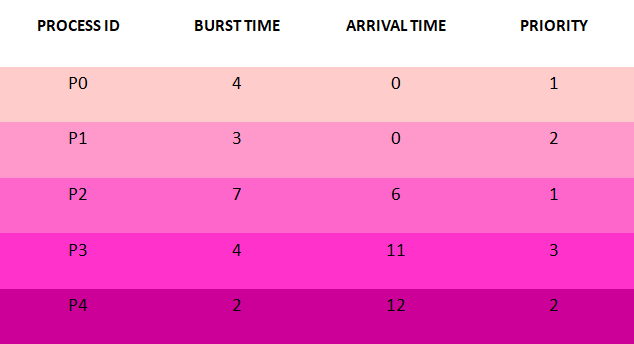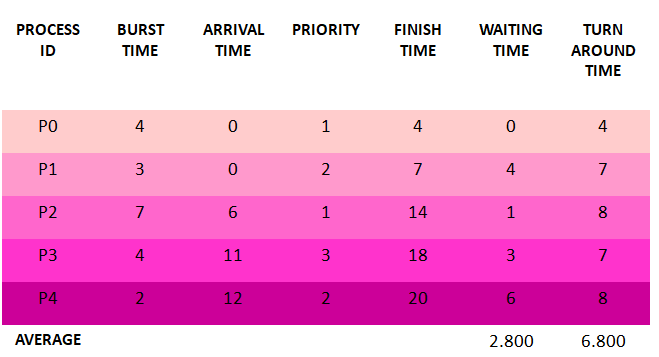×

Search anything:

# Non-Preemptive Priority CPU Scheduling Algorithm

#### Software Engineering AlgorithmsGet this book -> Problems on Array: For Interviews and Competitive Programming

Reading time: 30 minutes | Coding time: 15 minutes

Priority CPU Scheduling Algorithm is used to schedule the processes as per the priorities assigned to respective processes. Further this algorithm can be implemented in two parts Preemptive and Non-preemptive. Here we are majorly discussing about Non-preemptive Priority CPU Scheduling Algorithm.

In Non-preemptive Priority CPU Scheduling Algorithm, processes are scheduled as per the priorities assigned to respective task and next process is not schedule until and unless current execution of process is not completely finished.

### Algorithm

• Step 1 : Input the number of processes required to be scheduled using Non-Preemptive Priority Scheduling Algorithm, burst time for each process, arrival time and there respective scheduling priority.
• Step 2 : Using enhanced bubble sort technique, sort the all given processes in ascending order according to arrival time and if two or more processes having same arrival time then processes are sorted according to their priorities (low value represents high priority) in a ready queue.
• Step 3 : Calculate the Finish Time, Turn Around Time and Waiting Time for each process which in turn help to calculate Average Waiting Time and Average Turn Around Time required by CPU to schedule given set of processes.
• Step 3.1 : for i = 0, Finish Time T 0 = Arrival Time T 0 + Burst Time T 0
• Step 3.2 : for i >= 1, Finish Time T i = Burst Time T i + Finish Time T i - 1
• Step 3.3 : for i = 0, Turn Around Time T 0 = Finish Time T 0 - Arrival Time T 0
• Step 3.4 : for i >= 1, Turn Around Time T i = Finish Time T i - Arrival Time T i
• Step 3.5 : for i = 0, Waiting Time T 0 = Turn Around Time T 0 - Burst Time T 0
• Step 3.6 : for i >= 1, Waiting Time T i = Turn Around Time T i - Burst Time T i - 1
• Step 4 : Process with less arrival time (not necessary this process will have highest priority) comes first and gets scheduled first by the CPU.
• Step 5 : Calculate the Average Waiting Time and Average Turn Around Time.
• Step 6 : Stop.

### Example of Non-preemptive Priority CPU Scheduling Algorithm

Consider the following example containing five process with varied arrival time and priority values.• Step 1 : Processes get executed according to their arrival time and priority.

• Step 2 : Following shows the scheduling and execution of processes.

• Step 2.1 : At start P0 arrives and get executed because its arrival time is 0. Its duration of execution is 0-4 seconds.
System Time : 0
Process Scheduled : P0
Waiting Time : 4 – 4 = 0
Turn Around Time : 4 - 0 = 4

• Step 2.2 : P1 arrives at time 0 sec during which CPU was busy with Process P0, though there arrival timing are same scheduled as per their priority values. After completion of P3 , P2 is executed for duration 4-7 seconds.
System Time : 4
Process Scheduled : P0 P1
Waiting Time : 7 – 3 = 4
Turn Around Time : 7 – 0 = 7

• Step 2.3 : P2 arrives at time 6 sec but its execution is started at 7 sec after complete execution of process P1 , for a duration 7-14 seconds.
System Time : 7
Process Scheduled : P0 P1 P2
Waiting Time : 8 – 7 = 1
Turn Around Time : 14 – 6 = 8

• Step 2.4 : P3 arrives at time 11 sec but gets resources of CPU at 11th second for execution. Its execution period is 14-18 seconds.
System Time : 14
Process Scheduled : P0 P1 P2 P3
Waiting Time : 7 – 4 = 3
Turn Around Time : 18 – 11 = 7

• Step 2.5 : Similarly P4 arrives at time 12 sec but its execution gets started at time 18th second and last for a period 18-20 seconds.
System Time : 18
Process Scheduled : P0 P1 P2 P3 P4
Waiting Time : 8 – 2 = 6
Turn Around Time : 20 – 12 = 8

• Step 3 : After scheduling of all provided processes :### Implementation Explanation

• Step 1 : In following implementation, first the number of processes and their respective arrival ,burst timings and priorities are accepted by class method having following method signature.
``````void getProcessData(Scanner input)
``````
• Step 2 : After accepting the requried input for processes, method name priorityNonPreemptiveAlgorithm is called where, first the processes are sorted according there respective arrival time in ascending order (less arrival time high priority) and processes having same arrival timings are sorted according to their priorities (less value denotes high priority) using enhanced bubble sort method in class method having following signature.
``````void sortAccordingArrivalTimeAndPriority(int[] at,
int[] bt, int[] prt, String[] pid)
``````
• Step 3 : Further, after getting sorted according to arrival time and priority, finish, waiting, turn around timings are calculated in class method having following signature.
``````void priorityNonPreemptiveAlgorithm()
``````
• Step 3 : At last, the order of processes execution scheduled by Non-Preemptive Priority CPU Scheduling Algorithm is displayed as shown in Output Section.

### Implementation

Following is the implementation of Non-Preemptive Priority CPU Scheduling Algorithm in Java:

``````import java.util.Scanner;

public class NonPreemptivePriorityCPUSchedulingAlgorithm
{

int burstTime[];
int priority[];
int arrivalTime[];
String[] processId;
int numberOfProcess;

void getProcessData(Scanner input)
{
System.out.print("Enter the number of Process for Scheduling           : ");
int inputNumberOfProcess = input.nextInt();
numberOfProcess = inputNumberOfProcess;
burstTime = new int[numberOfProcess];
priority = new int[numberOfProcess];
arrivalTime = new int[numberOfProcess];
processId = new String[numberOfProcess];
String st = "P";
for (int i = 0; i < numberOfProcess; i++)
{
processId[i] = st.concat(Integer.toString(i));
System.out.print("Enter the burst time   for Process - " + (i) + " : ");
burstTime[i] = input.nextInt();
System.out.print("Enter the arrival time for Process - " + (i) + " : ");
arrivalTime[i] = input.nextInt();
System.out.print("Enter the priority     for Process - " + (i) + " : ");
priority[i] = input.nextInt();
}
}

void sortAccordingArrivalTimeAndPriority(int[] at, int[] bt, int[] prt, String[] pid)
{

int temp;
String stemp;
for (int i = 0; i < numberOfProcess; i++)
{

for (int j = 0; j < numberOfProcess - i - 1; j++)
{
if (at[j] > at[j + 1])
{
//swapping arrival time
temp = at[j];
at[j] = at[j + 1];
at[j + 1] = temp;

//swapping burst time
temp = bt[j];
bt[j] = bt[j + 1];
bt[j + 1] = temp;

//swapping priority
temp = prt[j];
prt[j] = prt[j + 1];
prt[j + 1] = temp;

//swapping process identity
stemp = pid[j];
pid[j] = pid[j + 1];
pid[j + 1] = stemp;

}
//sorting according to priority when arrival timings are same
if (at[j] == at[j + 1])
{
if (prt[j] > prt[j + 1])
{
//swapping arrival time
temp = at[j];
at[j] = at[j + 1];
at[j + 1] = temp;

//swapping burst time
temp = bt[j];
bt[j] = bt[j + 1];
bt[j + 1] = temp;

//swapping priority
temp = prt[j];
prt[j] = prt[j + 1];
prt[j + 1] = temp;

//swapping process identity
stemp = pid[j];
pid[j] = pid[j + 1];
pid[j + 1] = stemp;

}
}
}

}
}

void priorityNonPreemptiveAlgorithm()
{
int finishTime[] = new int[numberOfProcess];
int bt[] = burstTime.clone();
int at[] = arrivalTime.clone();
int prt[] = priority.clone();
String pid[] = processId.clone();
int waitingTime[] = new int[numberOfProcess];
int turnAroundTime[] = new int[numberOfProcess];

sortAccordingArrivalTimeAndPriority(at, bt, prt, pid);

//calculating waiting & turn-around time for each process
finishTime = at + bt;
turnAroundTime = finishTime - at;
waitingTime = turnAroundTime - bt;

for (int i = 1; i < numberOfProcess; i++)
{
finishTime[i] = bt[i] + finishTime[i - 1];
turnAroundTime[i] = finishTime[i] - at[i];
waitingTime[i] = turnAroundTime[i] - bt[i];
}
float sum = 0;
for (int n : waitingTime)
{
sum += n;
}
float averageWaitingTime = sum / numberOfProcess;

sum = 0;
for (int n : turnAroundTime)
{
sum += n;
}
float averageTurnAroundTime = sum / numberOfProcess;

//print on console the order of processes along with their finish time & turn around time
System.out.println("Priority Scheduling Algorithm : ");
System.out.format("%20s%20s%20s%20s%20s%20s%20s\n", "ProcessId", "BurstTime", "ArrivalTime", "Priority", "FinishTime", "WaitingTime", "TurnAroundTime");
for (int i = 0; i < numberOfProcess; i++) {
System.out.format("%20s%20d%20d%20d%20d%20d%20d\n", pid[i], bt[i], at[i], prt[i], finishTime[i], waitingTime[i], turnAroundTime[i]);
}

System.out.format("%100s%20f%20f\n", "Average", averageWaitingTime, averageTurnAroundTime);
}

public static void main(String[] args)
{
Scanner input = new Scanner(System.in);
NonPreemptivePriorityCPUSchedulingAlgorithm obj = new NonPreemptivePriorityCPUSchedulingAlgorithm();
obj.getProcessData(input);
obj.priorityNonPreemptiveAlgorithm();
}
}
``````

Input

``````Enter the number of Process for Scheduling           : 5
Enter the burst time   for Process - 0 : 4
Enter the arrival time for Process - 0 : 0
Enter the priority     for Process - 0 : 1
Enter the burst time   for Process - 1 : 3
Enter the arrival time for Process - 1 : 0
Enter the priority     for Process - 1 : 2
Enter the burst time   for Process - 2 : 7
Enter the arrival time for Process - 2 : 6
Enter the priority     for Process - 2 : 1
Enter the burst time   for Process - 3 : 4
Enter the arrival time for Process - 3 : 11
Enter the priority     for Process - 3 : 3
Enter the burst time   for Process - 4 : 2
Enter the arrival time for Process - 4 : 12
Enter the priority     for Process - 4 : 2
``````

Output

``````           Non-Preemptive Priority Scheduling Algorithm :
ProcessId           BurstTime         ArrivalTime            Priority          FinishTime         WaitingTime      TurnAroundTime
P0                   4                   0                   1                   4                   0                   4
P1                   3                   0                   2                   7                   4                   7
P2                   7                   6                   1                  14                   1                   8
P3                   4                  11                   3                  18                   3                   7
P4                   2                  12                   2                  20                   6                   8
Average            2.800000            6.800000
``````

### Complexity

The time and space complexity for Non-Preemptive Priority CPU Scheduling Algorithm :

• Worst case time complexity: `Θ(n2)`
• Average case time complexity: `Θ(n2)`
• Best case time complexity: `Θ(n)`
• Space complexity: `Θ(1)`

where N is the number of processes.

1. It is one of the simplest and easiest form of CPU Scheduling Algorithm.
2. Process having high priority doesn't have to wait for a long time.
3. It is used in the places where time varies eventually along with resources.

1. In this type of algorithm, process having highest priority compared to currently executing process have to wait till execution ends
2. Some low priority processes can be in waiting state for an indefinite time.
3. On system crash, low priority processes shall not be scheduled.
4. Resource Utilization in parallel is not possible here.

With this article at OpenGenus, you must have a complete idea of Non-Preemptive Priority CPU Scheduling Algorithm. Enjoy.